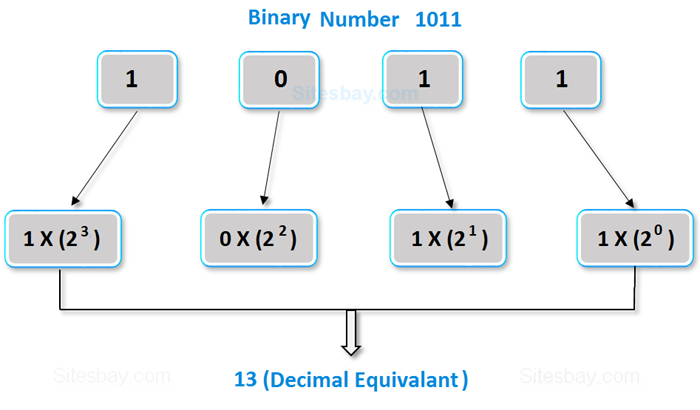# Convert Binary to Decimal in C

## Write a Program in C to Convert Binary to Decimal

In this types of program we takes a binary number as input and converts it into decimal number.• Take a binary number as input.
• Multiply each digits of the binary number starting from the last with the powers of 2 respectively.
• Add all the multiplied digits.
• Here total sum gives the decimal number.

## Convert Binary to Decimal in C

```#include<stdio.h>
#include<conio.h>

void main()
{
clrscr();
long int bin_number, dec_number=0, i=1, rem;
printf("Please Enter any Binary Number: ");
scanf("%ld",&bin_number);
while(bin_number!=0)
{
rem=bin_number%10;
dec_number=dec_number+rem*i;
i=i*2;
bin_number=bin_number/10;
}
printf("Equivalent Decimal value: %ld",dec_number);
getch();
}
```

## Output

```Please Enter any Binary Number: 11011
Equivalent Decimal value: 27
```

WIFI CCTV Camera